Select Page

# Arbitrary number fraction (arb.num.fr.)

Arb.num.fr.

### Definition in words

1. Arbitrary number fraction of component B in system 1 (ratio scale)
2. Difference between arbitrary number fraction of component B in system 1 in condition 1 and arbitrary number fraction of component B in system 1 in condition 0 (Differential scale)
3. Arbitrary number fraction of component B in system B compared with arbitrary number fraction of component B in reference system B (Ordinal scale)

Note 1:The concept has three definitions.

Note 2:The reference system is defined by the laboratory.

### Definition by equation

1.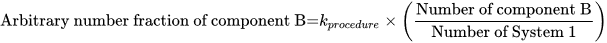2.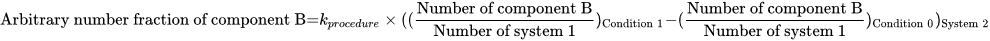Note: kprocedure=an arbitrary determined factor defined by the method procedure used.

### Result type

Ordinal scale
• Value set is ranked accoding to magnitude
• The value set and the limit of the value set are defined by the laboratory
• The value set can be either numerical values or words.

Example 1

Value set={none < some < high < very high}

Example 2

Value set={low < normal< high }

Example 3

Value set={0 < 1 < 2}

Example 4

Value set={negative < positive}

Example 5

Value set={+ < ++ < +++}

• Algebraic operations are not allowed (Ref. 2, 1.26)
• Possible comparison of values (a<b, a=b or a>b)
Rational scale
• Value sets are positive numerical values
• Numerical values from 0-∞
• Algebraic operations are allowed
• Possible comparison of values (a=nb; a≠nb)
Differential scale
• Value sets are numerical values on a linear differential scale
• Numerical values -∞ to ∞
• Values cannot be divided or multiplied
• Possible comprson of values (│a-b│=│c-d│;│a-b│≠│c-d│)

### Unit

International unit (IU) or procedure defined unit (p.d.u.) (non-SI units)

### Special rule(s) in the NPU syntax

“Procedure” is stated in the specification to the kind-of-property in the NPU definition.

“Procedure” indicates that the measurement procedure must be stated somewhere else than the NPU code and there may be difficulties in comparing values for the same NPU code.

If the ratio scale is attributed to the NPU definition, the unit can be non-SI unit.

If the unit is an International Unit (IU), an international certified reference material (e.g. WHO reference material) is specified in the specification to the kind-of-property.

If there exist no international certified reference material, the laboratory can specify the unit at the placeholder, procedure defined unit.

If ordinal scale is attributed, no unit is stated.

### Reference

1. Ferard G, Dybkaer R, Fuentes-Arderiu X. Compendium of Terminology and Nomenclature of Properties in Clinical Laboratory Sciences : Recommendations 2016. 1 ed: Royal Society of Chemistry; 2016. 182 p.
2. Mesures BIdPe. International Vocabulary of Metrology – Basic and General Concepts and Associated Terms VIM 3rd edition JCGM 200:2012 (JCGM 200:2008 with minor corrections). https://www.bipm.org: Bureau International des Poids et Mesures; 2008.

### Clinical Chemistry

Example 1 (ratio)
Generic abbreviated form NPU58645 Lkcs(Marrow)—Leukocytes(Carbohydrates); arb.num.fr.(proc.) = ?
Full form with a result NPU58645 Leukocytes(Marrow)—Leukocytes(Carbohydrates); arbitrary number fraction(procedure) = 0.2
Abbreviated form with a result NPU58645 Lkcs(Marrow)—Leukocytes(Carbohydrates); arb.num.fr.(proc.) = 0.2
Written expression The arbitrary number fraction of Leukocytes with Carbohydrates of all Leukocytes in Mr. Smith’s marrow is [0.2]. The measurement is performed  according to a procedure defined by the laboratory.
Equation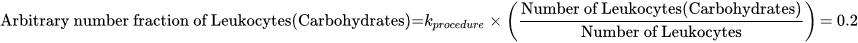### Clinical Immunology and Blood Banking

Example 2 (ratio)
Generic abbreviated form NPU56124 NK-lymcs(B)—NK-lymphocytes(CD107a); arb.num.fr.(K-562 induced; proc.) = ?
Full form with a result NPU56124 NK-lymphocytes(Blood)—NK-lymphocytes(CD107a); arbitrary number fraction(K-562 induced; procedure) = 0.7
Abbreviated form with a result NPU56124 NK-lymcs(B)—NK-lymphocytes(CD107a); arb.num.fr.(K-562 induced; proc.) = 0.7
Written expression The arbitrary number fraction of K-562 induced NK-lymphocytes with CD107a of all NK-lymphocytes in Mr. Smith’s Blood is [0.7]. The measurement is performed  according to a procedure defined by the laboratory.
Equation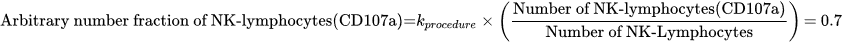Example 3 (ordinal)
Generic abbreviated form NPU53174 CD4-lymcs(B)—CD4-Positive T-Lymphocytes(CD40 ligand); arb.num.fr.(stim.; proc.) = ?
Full form with a result NPU53174 CD4-lymphocytes(Blood)—CD4-Positive T-Lymphocytes(CD40 ligand); arbitrary number fraction(stimulated; procedure) = ?
Abbreviated form with a result

NPU53174 CD4-lymcs(B)—CD4-Positive T-Lymphocytes(CD40 ligand); arb.num.fr.(stim.; proc.) = ?

Written expression The arbitrary number fraction of stimulated CD4-Positive T-Lymphocytes with CD40 ligand of all CD4-lymphocytes in Mr Smith’s blood is [normal], compared with the arbitrary number fraction of stimulated CD4-Positive T-Lymphocytes with CD40 ligand of all CD4-lymphocytes in a reference system. The measurement is performed according to a procedure defined by the laboratory,
Notes
1. The reference system is defined by the laboratory.
2. “High” is a value according to a value set defined by the laboratory.

### Clinical Microbiology

Example 4 (ordinal)
Generic abbreviated form NPU16379 Ercs(Marrow)—Trophozoite; arb.num.fr.(proc.) = none
Full form with a result NPU16379 Erythrocytes(Marrow)—Trophozoite; arbitrary number fraction(procedure) = none
Abbreviated form with a result NPU16379 Ercs(Marrow)—Trophozoite; arb.num.fr.(proc.) = none
Written expression The arbitrary number fraction of Trophozoite of erythrocytes in Mrs. Smith’s marrow is [none], compared with arbitrary number fraction of Trophozoite of erythrocytes in a reference system. The measurement is performed  according to a procedure defined by the laboratory.
Notes
1. The reference system is defined by the laboratory.
2. “None” is a value according to a value set defined by the laboratory.

### Molecular Biology and Genetics

Example 5 (differential)
Generic abbreviated form NPU53922 DNA fragment(P)—DNA fragment(Chromosome 21); arb.num.fr.(actual-norm; proc.) = ? (p.d.u.)
Full form with a result NPU53922 DNA fragment(Plasma)—DNA fragment(Chromosome 21); arbitrary number fraction(actual-norm; procedure) = 0.08
Abbreviated form with a result NPU53922 DNA fragment(P)—DNA fragment(Chromosome 21); arb.num.fr.(actual-norm; proc.) = 0.08
Written expression Difference between arbitrary number fraction of DNA fragment(Chromosome 21) of DNA fragment in Mrs. Smith’s plasma (actual) and arbitrary number fraction of DNA fragment(Chromosome 21) of DNA fragment in a reference system (norm) is [0.08 ]. The measurement is performed  according to a procedure defined by the laboratory.
Equation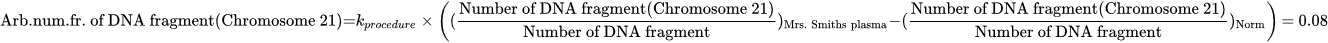### Reproduction and Fertility

Example 6 (ordinal)
Generic abbreviated form NPU58271 Spmzoa—Spermatozoa(agglutinated); arb.num.fr.(proc.) = ?
Full form with a result NPU58271 Spermatozoa—Spermatozoa(agglutinated); arbitrary number fraction(procedure) = 2
Abbreviated form with a result NPU58271 Spmzoa—Spermatozoa(agglutinated); arb.num.fr.(proc.) = 2
Written expression The arbitrary number fraction of agglutinated Spermatozoa of all Mr. Smith’s Spermatozoa is , compared with the arbitrary number fraction of agglutinated Spermatozoa of a reference system. The measurement is performed  according to a procedure defined by the laboratory.
Notes
1. The reference system is defined by the laboratory.
2. “2” is a value according to a value set defined by the laboratory.

### Reference

NPU database, https://www.ifcc.org/ifcc-scientific-division/sd-committees/c-npu/npusearch/ and http://www.labterm.dk/default.aspx

• # Kind-of-property

• ## Kind-of-quantity

### Reference

• Dybkaer R. Concept system on ‘quantity’: formation and terminology. Accredit Qual Assur. 2013;18(3):253-60.
• Dybkaer R. ISO terminological analysis of the VIM3 concepts ‘quantity’ and ‘kind-of-quantity’. Metrologia. 2010;47(3):127-34.
Date Term Note
1996-01-01

Arbitrary number fraction

Term established

QU50147